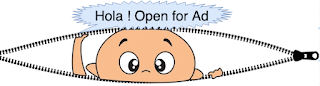## Latest Posts

Problem statement:- Traverse given binary tree alternatively left to right and right to left.In following diagram, LtoR represents : visit nodes from left to right in that level, similarly RtoL : visit nodes from right to left in that level. Read level order traversal of binary tree.

Zigzag level order traversal of above binary tree :- 12 18 23 11 43 12 27 98 32 78 56 87 29

Algorithm:-
In order to solve this problem, we can use two stacks,say currentLevelStack(CLS) and nextLevelStack(NLS). While traversing, we need to keep track of each level and for each level we use alternate stack.
1. Push root node into CLS and loop until this stack is not empty.
2. Pop an element from CLS and display, check whether we are traversing from left to right/right to left.
If left2right - push nodes into NLS left child followed by right child, otherwise right child followed by left child.
3. Do check for empty condition of CLS,if empty - it means we have traversed all nodes of that level. Swap references of CLS and NLS and toggle boolean flag left2right.
4. Repeat 2 and 3 until CLS is not empty and display nodes.
Sample code for zigzag level order traversal of binary tree in Java
```/* Zigzag traversal of binary tree - L2R and R2L alternatively */
public void zigzagLevelOrderTraversal(Node root) {
/* java.util.stack */
Stack<Node> currentLevelStack = new Stack<>();
Stack<Node> nextLevelStack = new Stack<>();
/* if true, current stack is used, else next stack */
boolean left2right = true;
if (root != null) {
currentLevelStack.push(root);
while (!currentLevelStack.isEmpty()) {
/* pop node from stack */
root = currentLevelStack.pop();
System.out.print(root.data + " ");
Node lc = root.leftChild;
Node rc = root.rightChild;
if (left2right) {
/* left child followed by right child */
if (lc != null) {
nextLevelStack.push(lc);
}
if (rc != null) {
nextLevelStack.push(rc);
}
} else {
/* Right child followed by left child */
if (rc != null) {
nextLevelStack.push(rc);
}
if (lc != null) {
nextLevelStack.push(lc);
}
}
/*
* Change order of traversal(L2R or R2L) and swap reference of
* current and next stack
*/
if (currentLevelStack.isEmpty()) {
left2right = !left2right;
/* swap reference of current and next stack */
Stack<Node> temp = currentLevelStack;
currentLevelStack = nextLevelStack;
nextLevelStack = temp;

}
}
}
}```
Explanation:- Extra space for two stack incurred (space complexity is order of O(n))and a boolean flag is used to keep track of we are traversing from left to right or right to left.As discussed in above, loop until currentLevelStack(CLS) is not empty and push all child nodes in nextLevelStack(NLS). Once stack is empty change the references of CLS and NLS (in order to avoid moving all elements from NLS to CLS). Boolean flag is also toggled to change direction of traversal in next level.

#### Complete sample program zigzag level order traversal of binary tree in Java

```package com.devinline.trees;

import java.util.Stack;

public class ZigzagBinaryTreeTraversal {
/* Zigzag traversal of binary tree - L2R and R2L alternatively */
public void zigzagLevelOrderTraversal(Node root) {
/* java.util.stack */
Stack<Node> currentLevelStack = new Stack<>();
Stack<Node> nextLevelStack = new Stack<>();
/* if true, current stack is used, else next stack */
boolean left2right = true;
if (root != null) {
currentLevelStack.push(root);
while (!currentLevelStack.isEmpty()) {
/* pop node from stack */
root = currentLevelStack.pop();
System.out.print(root.data + " ");
Node lc = root.leftChild;
Node rc = root.rightChild;
if (left2right) {
/* left child followed by right child */
if (lc != null) {
nextLevelStack.push(lc);
}
if (rc != null) {
nextLevelStack.push(rc);
}
} else {
/* Right child followed by left child */
if (rc != null) {
nextLevelStack.push(rc);
}
if (lc != null) {
nextLevelStack.push(lc);
}
}
/*
* Change order of traversal(L2R or R2L) and swap reference of
* current and next stack
*/
if (currentLevelStack.isEmpty()) {
left2right = !left2right;
/* swap reference of current and next stack */
Stack<Node> temp = currentLevelStack;
currentLevelStack = nextLevelStack;
nextLevelStack = temp;

}
}
}
}

public static void main(String[] args) {
ZigzagBinaryTreeTraversal zlo = new ZigzagBinaryTreeTraversal();
Node root = zlo.new BinaryTree().createTree();
System.out.print("Level order traversal in zigzag order: ");
zlo.zigzagLevelOrderTraversal(root);
}

class BinaryTree {
Node root;

public BinaryTree() {
root = null;
}

public Node createTree() {
if (root == null) {
root = new Node(12);
}
root.setLeftChild(new Node(23));
root.setRightChild(new Node(18));
root.getLeftChild().setLeftChild(new Node(11));
root.getLeftChild().setRightChild(new Node(43));
root.getRightChild().setRightChild(new Node(27));
root.getRightChild().setLeftChild(new Node(12));
root.getLeftChild().getLeftChild().setLeftChild(new Node(56));
root.getLeftChild().getLeftChild().setRightChild(new Node(78));
root.getRightChild().getLeftChild().setRightChild(new Node(98));
root.getRightChild().getLeftChild().setLeftChild(new Node(32));
root.getLeftChild().getLeftChild().getRightChild()
.setLeftChild(new Node(87));
root.getRightChild().getLeftChild().getRightChild()
.setLeftChild(new Node(29));
return root;
}
}

class Node {

private int data;
private Node leftChild;
private Node rightChild;

public Node(int data) {
this.data = data;
leftChild = null;
rightChild = null;
}

public int getData() {
return data;
}

public void setData(int data) {
this.data = data;
}

public Node getLeftChild() {
return leftChild;
}

public void setLeftChild(Node leftChild) {
this.leftChild = leftChild;
}

public Node getRightChild() {
return rightChild;
}

public void setRightChild(Node rightChild) {
this.rightChild = rightChild;
}
}
}```
========Sample output===============
Level order traversal in zigzag order: 12 18 23 11 43 12 27 98 32 78 56 87 29
==================================

#### 1 comment:

1.